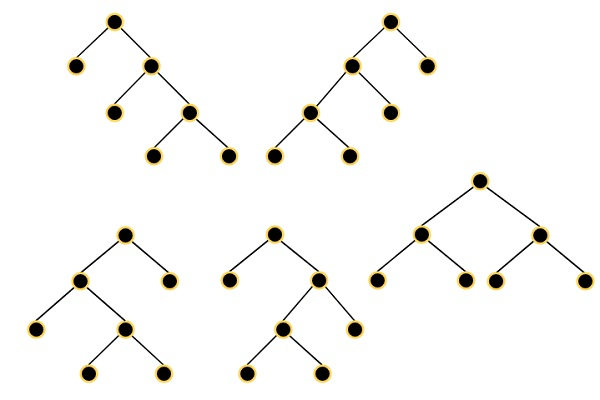# All Possible Full Binary Trees in C++

Suppose a full binary tree is a binary tree where each node has exactly 0 or 2 children. So we have to find a list of all possible full binary trees with N nodes. Each node of each tree in the answer must have node.val = 0. The returned trees can be in any order. So if the input is 7, then the trees are −To solve this, we will follow these steps −

• Define a map m of integer type key and tree type value.

• define a method called allPossibleFBT(), this will take N as input

• is N is 1, then create a tree with one node whose value is 0, and return

• if m has the key N, then return m[N]  Define an array called temp, and req := N – 1

• for left in range 1 to req – 1

• right := req – left

• if left = 2 or right = 2, then go for next iteration

• leftPart := allPossibleFBT(left), rightPart := allPossibleFBT(right)

• for j in range 0 to size of leftPart - 1

• for k in range 0 to size of rightPart – 1

• root := a new node with value 0

• left of root := leftPart[j], right of root := rightPart[k]

• insert root into ans

• set m[N] := ans and return.

## Example(C++)

Let us see the following implementation to get a better understanding −

Live Demo

#include <bits/stdc++.h>
using namespace std;
class TreeNode{
public:
int val;
TreeNode *left, *right;
TreeNode(int data){
val = data;
left = right = NULL;
}
};
void tree_level_trav(TreeNode*root){
if (root == NULL) return;
cout << "[";
queue<TreeNode *> q;
TreeNode *curr;
q.push(root);
q.push(NULL);
while (q.size() > 1) {
curr = q.front();
q.pop();
if (curr == NULL){
q.push(NULL);
} else {
if(curr->left)
q.push(curr->left);
if(curr->right)
q.push(curr->right);
if(curr == NULL || curr->val == 0){
cout << "null" << ", ";
} else {
cout << curr->val << ", ";
}
}
}
cout << "]"<<endl;
}
class Solution {
public:
map < int, vector <TreeNode*> > m;
vector<TreeNode*> allPossibleFBT(int N) {
if(N == 1){
vector <TreeNode*> temp;
TreeNode *n = new TreeNode(1);
n->left = new TreeNode(0);
n->right = new TreeNode(0);
temp.push_back(n);
return temp;
}
if(m.count(N))return m[N];
vector <TreeNode*> ans;
int required = N - 1;
for(int left = 1; left < required; left++){
int right = required - left;
if(left == 2 || right == 2)continue;
vector <TreeNode*> leftPart = allPossibleFBT(left);
vector <TreeNode*> rightPart = allPossibleFBT(right);
for(int j = 0; j < leftPart.size(); j++){
for(int k = 0; k < rightPart.size(); k++){
TreeNode* root = new TreeNode(1);
root->left = leftPart[j];
root->right = rightPart[k];
ans.push_back(root);
}
}
}
return m[N] = ans;
}
};
main(){
vector<TreeNode*> v;
Solution ob;
v = (ob.allPossibleFBT(7)) ;
for(TreeNode *t : v){
tree_level_trav(t);
}
}

## Input

7

## Output

[1, 1, 1, null, null, 1, 1, null, null, 1, 1, null, null, null, null, ]
[1, 1, 1, null, null, 1, 1, 1, 1, null, null, null, null, null, null, ]
[1, 1, 1, 1, 1, 1, 1, null, null, null, null, null, null, null, null, ]
[1, 1, 1, 1, 1, null, null, null, null, 1, 1, null, null, null, null, ]
[1, 1, 1, 1, 1, null, null, 1, 1, null, null, null, null, null, null, ]